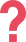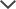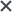## Cognitive Abilities Test Non-Verbal section

The CogAT Nonverbal Battery is used to assess a student’s reasoning abilities through the use of spatial and figural content. The test is useful for assessing the development of students who have trouble with reading, limited capability in English, or who have limited opportunities.

The subtests use geometric shapes and figures which have unlikely been seen by the students in their formal schooling. There is no reading required among the questions in the CogAT Nonverbal test. These three subtests are: Figure Matrices, Paper Folding, and Figure Classification. These three subtests combined make up the CogAT Nonverbal score.

## Number of Items by Level & Section

Nonverbal Battery Level 5/6 Level 7 Level 8 Level 9 Level 10-18
Figure Matrices 14 16 18 20 22
Paper Folding 10 12 14 16 16
Figure Classification 14 16 18 20 22

## Figure Matrices

Each question in this subsection portrays a 2 x 2 matrix. As in the Picture/Verbal Analogies in the CogAT Verbal Battery and the Number Analogies in the CogAT Quantitative Battery, the students must determine the relationship between the pictures in the two boxes in the top row and apply the same relationship in the bottom row. However, the Figure Matrices utilizes spatial forms. In all level, students are presented with pictures in the matrices. This sub-tests presents a relationship that is not always clear. Therefore, developing the skill to decipher the relationship is crucial to solve these types of questions. Below, you can see an example of a Figure Matrices question from a Level 9 CogAT test:The correct answer is B. In the above analogy, as you move across the rows, the figures rotate clockwise 90 degrees (1/4 of a circle). Additionally, the colors of the figures are inversed. Parts of the figure which are white become black and parts of the figure which are black become white. The answer will exhibit the above analogous properties.

Tip: It is sometimes helpful to look at one part of the figure. For example, look at the single square in the top analogy. Observe how it rotates 1/4 of a circle in a clockwise direction and changes colors from black to white.

## Paper Folding

For Level 5/6 - Level 8, students must determine how a piece of paper will look once folded. In Levels 9 and up, the questions may include hole punching and require students to determine how the paper will appear when it is unfolded. This sub-test measures the spatial capabilities of a student. The ability to unfold the paper and foresee the resulting image can be indicative of a strong analytical mind. Below, you can see an example of a Paper Folding question from a Level 10 CogAT test:The correct answer is C. First the paper was folded diagonally from the upper-left corner to the lower-right corner. Then it was folded again from the lower-left corner to the upper-right corner. Then three holes in different shapes were punched out. Therefore, when the paper is unfolded the holes will mirror each other across the diagonals of the page like this:To solve this question look for the answer choices in which the holes do not mirror each other in the right way. Answer choices B and D can be eliminated in this way. Next, focus on the area of each remaining answer choice that appears in the rightmost frame of the question series (in this case the triangular area on the right side) and eliminate all choices that are different. Answer choice A can be eliminated as the circular hole is under the pentagon hole but should be above it. E can also be eliminated as the pentagon has been rotated. We are left with C as the only correct answer.

## Figure Classification

Like the Picture/Verbal Classification in the CogAT Verbal Battery, students are presented with three figures and the student is to select the answer choice that is supposed to be the fourth figure of the set by determining how the figures and shapes are similar. The idea is to develop the skill of recognizing patterns and applying them to make a smart choice. Below, you can see an example of a Figure Classification question from a Level 8 CogAT test:The correct answer is A. In this question the three figures are all circles. The only answer choice that is a circle is A. The rest of the answers are all shapes that are different than the shapes in the question. Therefore, A is the correct answer.

Access More CogAT Questions Like These!

Need HelpNeed Help